# Polarity

(diff) ← Older revision | Latest revision (diff) | Newer revision → (diff)

polar transformation

A correlationfor which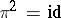, that is,if and only if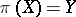. A polarity divides all subspaces into pairs; in particular, if a pair is formed by the subspaces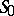and, where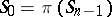is a point andis a hyperplane, thenis called the pole of the hyperplaneand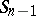is called the polar of the point. A projective space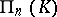over the skew-fieldhas a polarity if and only ifadmits an involutory anti-automorphism(that is,). Suppose thatis represented by a semi-bilinear form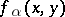. Thenis a polarity if and only if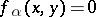implies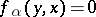.

A polarityis either a symplectic correlation, characterized by the fact that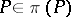for every point(in this case,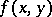is a skew-symmetric form on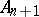, whileis a field), orcan be represented as an-symmetric form on: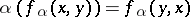(a symmetric polarity), in this case the existence of a non-strictly isotropic null subspace is equivalent to the fact that the characteristic of the skew-field equals 2 (in particular, if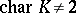, then any null subspace is strictly isotropic).

Relative to a polarityone defines decomposition of a projective space into subspaces, which makes it possible to reduce the semi-bilinear form representingto canonical form. The most important among these are the following:— a maximal non-isotropic null subspace; its dimension is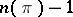, whereis even and is called the deficiency of, andis skew-symmetric;— a maximal strictly-isotropic subspace; its dimension is,is called the index,;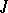— a component, free or null subspace, non-isotropic, whereis positive or negative definite,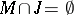.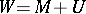— a maximal null subspace; its dimension is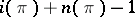.

A projective transformationis called-admissible (relative to the polarity) if. A semi-linear transformationinduces a-admissible projective transformation if and only if inthere is afor which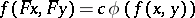. The-admissible transformations form a group,(called the polarity group). If the groupis transitive, either every point of the space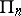is null (and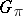is called symplectic) or there is no null point (and in this caseis called orthogonal for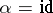, and unitary for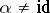).

How to Cite This Entry:
Polarity. Encyclopedia of Mathematics. URL: http://encyclopediaofmath.org/index.php?title=Polarity&oldid=15854
This article was adapted from an original article by M.I. Voitsekhovskii (originator), which appeared in Encyclopedia of Mathematics - ISBN 1402006098. See original article